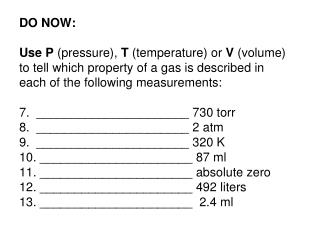DownloadDownload PresentationCombine gas law

Combine gas law

Download PresentationCombine gas law

- - - - - - - - - - - - - - - - - - - - - - - - - - - E N D - - - - - - - - - - - - - - - - - - - - - - - - - - -
Presentation Transcript

1. DO NOW:Use P (pressure), T (temperature) or V (volume) to tell which property of a gas is described in each of the following measurements:7. ______________________ 730 torr8. ______________________ 2 atm9. ______________________ 320 K10. ______________________ 87 ml11. ______________________ absolute zero12. ______________________ 492 liters13. ______________________ 2.4 ml

2. Combine gas law • STP = standard temperature and pressure • ST = standard temp = 273K • SP = standard pressure = 1 atmor 101.3 kPa

3. Step 1 • if temperature is not in K, convert! • K = C + 273 • C = K - 273

4. Step 2: Plug the numbers into the equation PV PV T T =

5. Step 3: Solve 80 ml x 96 kPa V x 99 kPa 300 K 273K = 80 x 96 x 273 = V x 99 x 300 80 x 96 x 273 = V (99 x 300)